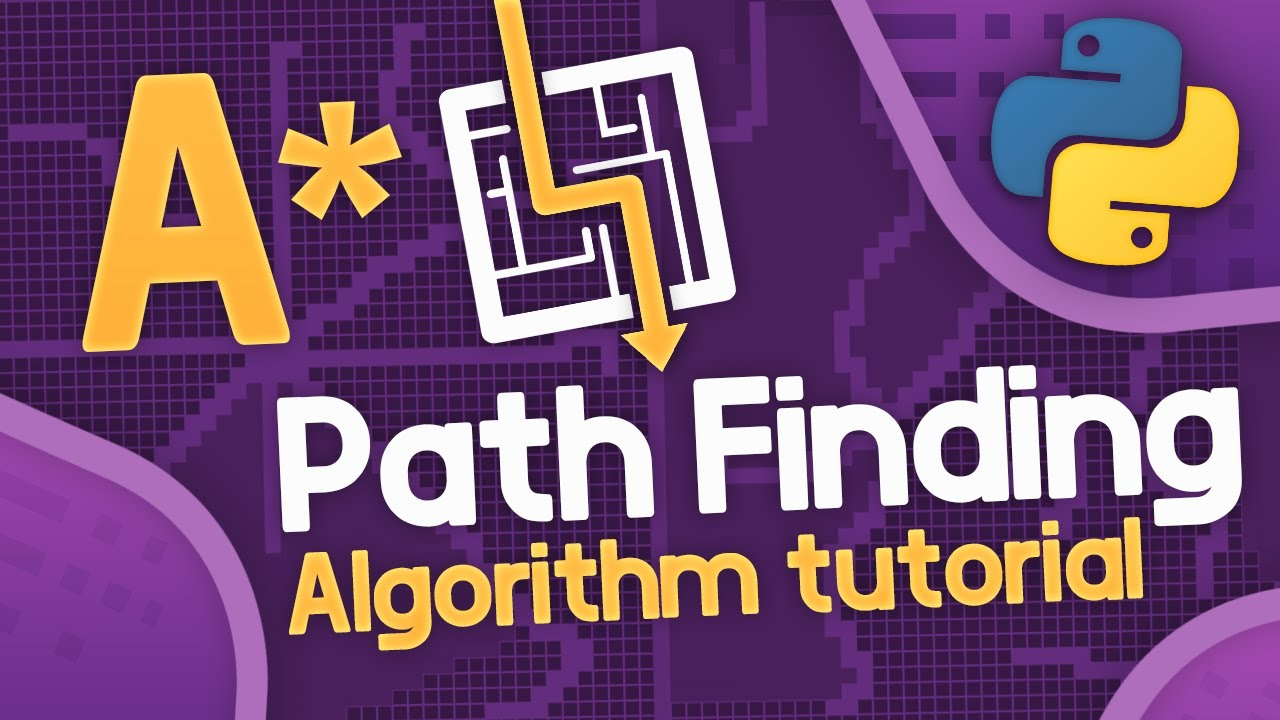# A Pathfinding Visualization Tutorial - Python A Path Finding TutorialThis A* Path Finding tutorial will show you how to implement the a* search algorithm using python. We will be building a path finding visualizer tool to visualize the a star pathfinding algorithm as it runs. This astar pathfinding algorithm is an informed search algorithm which means it is much more efficient that your standard algorithms like breadth first search or depth first search.

This A* Path Finding tutorial will show you how to implement the a* search algorithm using python. We will be building a path finding visualizer tool to visualize the a star pathfinding algorithm as it runs. This astar pathfinding algorithm is an informed search algorithm which means it is much more efficient that your standard algorithms like breadth first search or depth first search.

⭐️ Timestamps ⭐️
00:00:00 Introduction
00:03:17 - Algorithm Explanation
00:19:22 - Implementation

⚡ Please leave a LIKE and SUBSCRIBE for more content! ⚡

⭐ Tags ⭐

• Tech With Tim
• A* Pathfinding
• A Star Path Finding Tutorial
• A Star Search Algorithm
• Path Finding A Star Tutorial
• Python Path Finding Tutorial
• Python A Star Path Finding Algorithm
• Path Finding Visuallizer

⭐ Hashtags ⭐
#python #pathfinding #astar